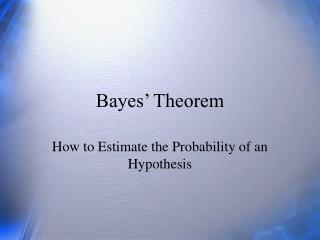DownloadDownload PresentationBayes’ Theorem

# Bayes’ Theorem

Télécharger la présentation## Bayes’ Theorem

- - - - - - - - - - - - - - - - - - - - - - - - - - - E N D - - - - - - - - - - - - - - - - - - - - - - - - - - -
##### Presentation Transcript

1. Bayes’ Theorem How to Estimate the Probability of an Hypothesis

2. Questions • What is Bayes’ theorem? • What can you do with Bayes theorem? Why might it be useful?

3. Bayes Theorem (1) Bayesian statistics are about the revision of belief. Bayesian statisticians look into statistically optimal ways of combining new information with old beliefs. Prior probability – personal belief or data. Input. Likelihood – likelihood of data given hypothesis. Posterior probability – probability of hypothesis given data. Scientists are interested in substantive hypotheses, e.g., does Nicorette help people stop smoking. The p level that comes from the study is the probability of the sample data given the hypothesis, not the probability of the hypothesis given the data. That is

4. Bayes Theorem (2) Bayes theorem is old and mathematically correct. But its use is controversial. Suppose you have a hunch about the null (H0) and the alternative (H1) that specifies the probability of each before you do a study. The probabilities p(H0) and p(H1) are priors. The likelihoods are p(y| H0) and p(y| H1). Standard p values. The posterior is given by: p(H1|y)=1-p(H0|y)

5. Bayes Theorem (3) Suppose before a study is done that the two hypotheses are H0: p =.80 and H1: p=.40 for the proportion of male grad students. Before the study, we figure that the probability is .75 that H0 is true and .25 That H1 is true. We grab 10 grad students at random and find that 6 of 10 are male. Binomial applies.

6. Bayes Theorem (4) Bayes theorem says we should revise our belief of the probability that H0 is true from .75 to .70 based on new data. Small change here, but can be quite large depending on data and prior. Problems with choice of prior. Handled by empirical data or by “flat” priors. There are Bayesian applications to more complicated situations (e.g., means and correlations). Not used much in psychology except in meta-analysis (empricial Bayes estimates) and judgment studies (Taxis, etc).

7. Review • What is the main point of Bayes theorem? • What can you do with Bayes theorem? Why might it be useful?